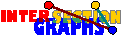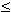# Random Models

It is certainly convincing to consider intersection graphs of random models, but one has to be careful in defining what a random set (of a certain shape) should be. For instance, how would you define a random interval, or a random unit disk? The given distribution should also make sense, for instance in applications.

For instance, a model for random interval graphs has been proposed in [JSW90]. Choose n pairs of points, which then are the endpoints of the intervals, from a given continuous distribution on the line. It is easy to see that this distribution actually doesn`t matter. The authors derived interesting properties of the resulting random interval graph, namely that the expected clique size is n/2, the expected independence number 2 sqrt(n pi), and the resulting graph has some vertex adjacent to all others with probability 2/3. Thus in most cases we get diameter 2.Are there other reasonable models for random interval graphs? What about the model for random unit interval graphs, where we choose n unit intervals out of [0,m], with uniform distribution?

Like general random graphs, intersection graphs of random models seem to have very sharp features, as can be seen in the example above. Another example is the following: Among the set of all 2N subsets of {1,2, ... , N}, choose n(N) independent random subsets. Then the intersection graphs G(n.N) have the following properties:[M91]: For the model described above, if n <<(4/3) N/2, then G(n,N) is complete almost surely, if (4/3) N/2 << n << 2N, then G(n,N) is connected with diameter 2, almost surely, and if 2N << n, then G(n,N) contains isolated vertices almost surely.

Thus this model wouldn`t be useful for getting connected graphs of large diameter, for instance. It should be worthwhile to try intersection graphs of which models have which properties.

### A recognition hierarchy

There are cases where we only partially succeed in recognition. Some times we have to require large enough minimum degree (i.e. minimum |a*|) for the model, sometimes we can handle all graphs of large enough minimum degree.

A result of the latter shape is surely preferable, since if we require large enough minimum degree in the model, then we also get large minimum degree in the graph. Now every result requiring large minimum degree of the hypergraph works in almost all cases in most models, so we get the following hierarchy, going from weak to strong results:

1. Recognition in almost all cases.
2. Recognition for models of high enough minimum degree.
3. Recognition for graphs of high enough minimum degree.
4. Recognition in all cases.

For intersection graphs of k-uniform hypergraphs, k>2 , as well as for k-facet graphs, k>2 , I do not know about results of level 2. But level 1 can be achieved:[P**]: Let 1t< k be integers, and let p=p(n) >> n^{-1/2+}, for>0. Then there is an algorithm that recognizes almost all t-intersection graphs of random hypergraphs Hk(n,p) in polynomial time.

You may see a sketch of the proof for facet graphs here.

Back to the start page for intersection graphs.

Erich Prisner